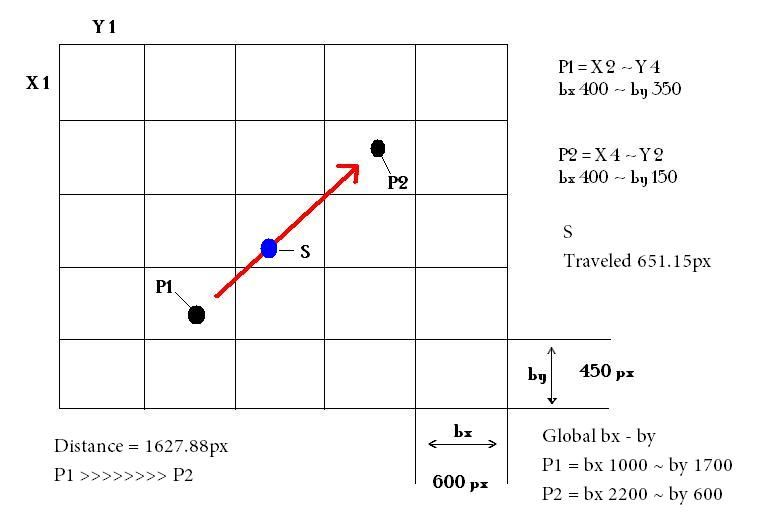# Need some help from a math genius

## Recommended Posts

I'm completly lost on how to solve this. The first theory i had didnt work lol :PSorry if this is in the wrong spot in the forum. Seems to be no math topicI"m some what decent with trionometry but i just cant figure out how to solve it. Made a picture to show what im trying to doS is moving between P1 and P2I need to find the global location of Swhich is the bx and byReally hope someone knows how to do this##### Share on other sites

Are you saying that you have the positions of P1 and P2, and you know how far S is from P1, and you want to calculate the position of S in the larger coordinate system?

##### Share on other sites

Hey justsomeguy Ya thats what im saying S is 40 percent towards P2 I just realized though that all i have to do is just add 40 percent to P1's global bx and subtract 40 percent from P1's global by and that will give me its global position but if it was going the oppisite direction it would be reversesubtract from P1's global bx and add to P1's global by so my problem is geometry not trig I guess so idk i could figure out a statement to tell rather or not to subtract or add

##### Share on other sites

If you know the distance from P1 to S, then that length is C in the Pythagorean theorem. Not only do you know that length, but you also know (or can calculate) the angle of that line between P1 and P2 that S is traversing. With the length of C and the angle, you can calculate the lengths of A and B, the other 2 sides of the right triangle that P1 and S are points of. A and B would be the X and Y offsets of S from P1, so you can add (or subtract, depending on where the origin is in that coordinate system) those to the position of P1 to get the position of S.

##### Share on other sites

I tried a concept i came up with first that works.going to look into the angle idea now though Its wierd that the trig book i have doesn't have the forumla that you are talking about in it. angle = tan inverse ( X / Y) .... not sure what x/y i use on that equation though. anyways the way i figured it out is with out even using trig.. accept for finding the percent traveled bx = P1_bx + ((P2_ bx - P1_bx) * percent traveled which was 40 percent)by = P1_by + ((P2_by - P1_by) * percent traveled which was 40 percent) so filling in the numbers would be bx = 1000 + ((2200 - 1000) * .40)by = 1700 + ((600 - 1700) * .40) return S is located atbx = 1480 pxby = 1260 px

##### Share on other sites

angle = tan inverse ( X / Y) .... not sure what x/y i use on that equation though.
If you have a right triangle, where the sides are A, B, and C, where C is the hypoteneuse, then you can get the angle between C and A by taking the arctangent of B / A, where B is the side along the Y axis and A is the side along the X axis. There's a diagram here: http://www.mathwords.com/s/sohcahtoa.htm